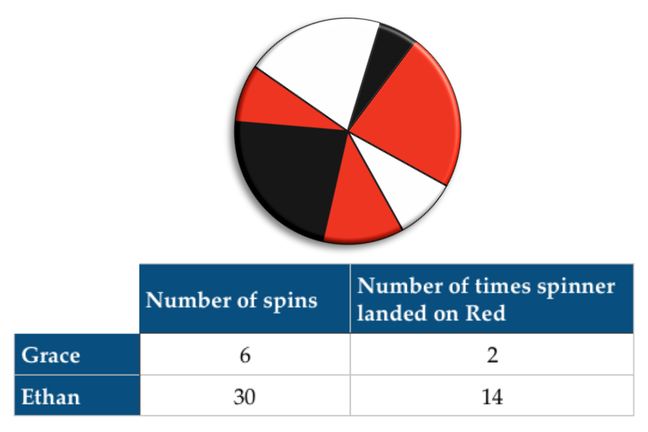## Want to keep learning?

This content is taken from the Cambridge University Press's online course, Teaching Probability. Join the course to learn more.
3.7

## Cambridge University PressSpinner and data for question 2

# Expectation and experiments

In this step we continue our exploration of examination topics in probability.

Our next topics are:

• Expectation

• Estimating probabilities from experiments

Our approach for these topics will be a little different. As before, we will introduce our topics, set some sample examination-style questions, and provide sample solutions. However, rather than simply inviting you to share your preferred teaching approaches, we are going to show you how a spreadsheet model can be used to simulate data and reinforce the key learning points for the topic.

We have used spreadsheet models before, but we have presented these ‘ready made’. In the next step, we will build up a simple spreadsheet model, step-by-step. After you have seen how this model is built up, we can use it to explore one of the sample examination questions. Our intention is to enable you to develop your own simulations of random processes.

First, though, let us turn to our new topics.

### Expectation

Expectation is the expected frequency of an event, given its probability and the number of trials that take place. The expected frequency of an event is the product of its probability and the number of trials that take place.

One way of defining (experimental) probability of an event is as the limiting value of the relative frequency in a long series of trials. If the event we are interested in occurs with frequency f in n trials, then we can define the probability p of our event with the formula:

$p=\lim _{ n\rightarrow \infty }{ \left( \frac { f }{ n } \right) }$

More simply, the estimated probability from n trials is given by the formula:

$p=\frac{f}{n}$

– where the estimate gets better as n increases.

The formula for expectation is essentially a rearrangement of this to produce $$f=np$$. Notice that we need to reverse the order of our reasoning here - the original formula defined probability in terms of the results of an experiment, while we now want to predict results from a given value of probability. In recognition of this, we refer to the expected frequency or ‘expectation’, rather than an actual frequency.

### Estimating probabilities from experiments

We have already seen how relative frequency can be used as an estimate of probability: the estimated probability of an event p that occurs with frequency f in n trials is given by the formula $$p=\frac{f}{n}$$. Examination questions often check that candidates understand that estimates of probability become more reliable as the number of trials increases.

## Sample examination-style questions

### Question 1

Oliver flips a fair coin 200 times.

• How many times does he expect to get a Head?

• Oliver gets 110 Heads and claims the coin is not fair because it has a bias towards getting Heads. Is this claim reasonable?

### Question 2

Grace and Ethan used a spinner with sections coloured red, white, and black to produce the data shown in the table. [See illustration above.]

They want to estimate the probability of the spinner landing on Red.

• Grace says: ‘There are three possible outcomes, and so the probability of landing on Red is $$\frac{1}{3}$$. And my results prove it!’ What is wrong with Grace’s opinion?

• Ethan says: ‘The probability of landing on Red is $$\frac{14}{30}$$.’ Why is this a more reliable estimate than Grace’s?

• What might be a better estimate than $$\frac{14}{30}$$?